﻿ Applying the Successive Over-relaxation Method to a Real World Problems

### Applying the Successive Over-relaxation Method to a Real World Problems

T. Mayooran, Elliott Light

American Journal of Applied Mathematics and Statistics

## Applying the Successive Over-relaxation Method to a Real World Problems

T. Mayooran1,, Elliott Light1

1Department of Mathematics and Statistics, Minnesota state university, Mankato, USA

### Abstract

Solving a system of equations by Ax=b, where A is a n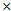n matrix and b and n1 vector, can sometime be a daunting task because solving for x can be difficult. If you were given an algorithm that was efficient, that’s great! What if you could make it solve the problem even faster? That’s even better. We will first take a look at establishing the basics of the successive over-relaxation method (SOR for short), then we’ll look at a real-world problem we applied the SOR method to, solving the heat-equation when a constant boundary temperature is applied to a flat plate.

• T. Mayooran, Elliott Light. Applying the Successive Over-relaxation Method to a Real World Problems. American Journal of Applied Mathematics and Statistics. Vol. 4, No. 4, 2016, pp 113-117. http://pubs.sciepub.com/ajams/4/4/3
• Mayooran, T., and Elliott Light. "Applying the Successive Over-relaxation Method to a Real World Problems." American Journal of Applied Mathematics and Statistics 4.4 (2016): 113-117.
• Mayooran, T. , & Light, E. (2016). Applying the Successive Over-relaxation Method to a Real World Problems. American Journal of Applied Mathematics and Statistics, 4(4), 113-117.
• Mayooran, T., and Elliott Light. "Applying the Successive Over-relaxation Method to a Real World Problems." American Journal of Applied Mathematics and Statistics 4, no. 4 (2016): 113-117.

 Import into BibTeX Import into EndNote Import into RefMan Import into RefWorks

1
Prev Next

### 1. Introduction

Successive over-relaxation (SOR) is one of the most important method for solution of large linear system equations. It has applications in Fluid Dynamics, mathematical programming, linear elasticity and machine learning etc. The examples of applications of SOR in Dynamics include study of steady heat conduction, turbulent flows, boundary layer flows or chemically reacting flows. For this reason, SOR method is important for both researchers and business policymakers.

In the real world, time is always something valuable, something no one wants to waste; when it comes to solving systems of equations, it can sometimes be better to get a close approximation of the solution than to get the exact solution for this very reason, among others. This is where the successive over-relaxation method (SOR) can come into play. The industry standard for finding exact methods, Gaussian elimination, requires approximatelyoperations to solve the system, which becomes time consuming when n gets big. SOR on the other hand, while only giving us an approximation, can give us these approximations much faster than Gaussian elimination can. SOR was developed in 1950 by David Young and H. Frankel in 1950 and was developed to be used on digital computers. It was developed by modifying the Gauss-Seidel iteration model. The Gauss-Seidel model is based on the following steps.

1. Given Ax = b. where A and b are known and an initial guess for x, x0

2.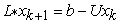Where L* is the lower triangular components of matrix A, U is the upper triangular components of A, b is our b vector and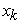is the kth approximation of x and xk+1 is the next iteration of x.For the numerical solution of the accelerated Overrelaxation method was introduced by Hadjidimos in  and is a two-parameter generalization of the successive Overrelaxation (SOR) method.The SOR method works this way.

1. Given Ax = b where A and b are known, x unknown, and an initial guess for x, x0

2. Letwhere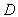is the main diagonal of A, L the lower triangle components of A and U the upper triangle components of A.

3.Whereis the kth approximation of,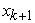is the next iteration of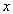,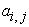is the corresponding element of matrix A, b is our vector and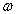is our relaxation factor. We’ll talk more about selecting an appropriate relaxation factor when we get to the next section, but for now, note that if, we get the Gauss-Siedel method. The convergence is enhanced because the value at a particular iteration is made up of a combination of the old value and the newly calculated value, namely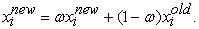The SOR method is very similar to the Gauss-Seidel method except that it uses a scaling factor to reduce the approximation error. Consider the following set of equationsFor Gauss-Seidel method, the values at the k iteration are given by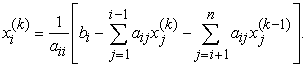It should be noted that for the calculation of xi, the variables with index less than i are at the (k) iteration while the variables with index greater than i are at still at the previous (k-1) iteration. The equation for the SOR method is given asThe term in the bracket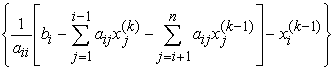is just difference between the variables of the previous and present iterations for the Gauss-Seidel methodThis difference is essentially the error for the iteration since at convergence this difference must approach zero. The SOR method obtains the new estimated value by multiplying this difference by a scaling factorand adding it to the previous value. The SOR equation can also be written in the following formWhen= 1 the above equation is the formula for Gauss-Seidel method, when< 1 it is the under-relaxation method, and when< 1 it is the over-relaxation method. We use the SOR method to solve the set of equations presented in heat problem.

Figure 1 shows the number of iterations required for convergence as a function of the scaling factor. There is a minimum in the number of iterations atof about 1.2. Normally the value of the scaling factor for a minimum iteration is between 1 and 2 and this value cannot be determined beforehand except for some special cases. Under-relaxation method (< 1) always requires more iterations than the Gauss-Seidel method. However under-relaxation is sometimes used to slow the convergence if a value of the scaling factor ≥ 1 leads to divergence.Download asVeiw figureFigures index
Figure 1. The variation of number of iterations with scaling factor

### 2. Algorithm

To solvegiven the parameterand an initial approximation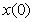:

INPUT the number of equations and unknowns n; the entries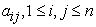, of the matrix A; the entriesof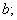the entriesofthe parameter; tolerance TOL; maximum number of iterations N.

OUTPUT the approximate solution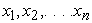a message that the number of iterations was exceeded.

Step 1 Set.

Step 2 While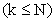do Steps 3–6.

Step 3 ForSet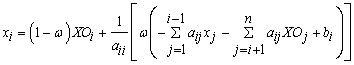Step 4 If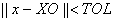then OUTPUT(The procedure was successful.) STOP.

Step 5 Set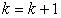.

Step 6 For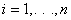set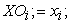Step 7 OUTPUT (‘Maximum number of iterations exceeded’);

(The procedure was successful.) STOP.

### 3. Application of SOR

Now let’s move to our application of SOR. The application utilizes the heat equation, which is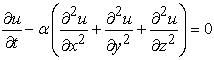Which simply states that as time increases, t, the temperature,, and changes over the three special coordinates,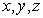where alpha is a positive constant. For our example, we’re only going to use two dimensions,and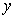. Let us move to that example. Utilizing an article by Ronal S. Besser we picked and modified his first simple example, the constant boundary temperature example. We are given a 0.9 x 0.9 m plate and its sides are heated to 273 Kelvin. See the model below for the full, initial set up.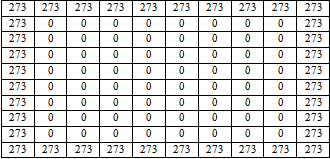The value at each entry in the matrix represents the temperature at that position, aka a node, on the plate. To solve this system, we used the finite difference method. The FDM utilizes some large but finite about of rectangular elements such thatWhereand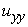are the temperatures at the points x and y respectfully. We can rewrite the FDM equation like soand rewriting it and multiplying everything by -1, we getTo set the matrix system up, first we need to establish outand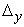values. For this, we set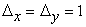.

Our coefficient matrix A2, comes from the inner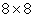matrix of zeros from the bigger matrix A, and b will be our vector of known values surrounding the nodal points of A2. To find our values, we start at the A22,j and add up the values around the node. If the value is known, it is put into the b vector, if it is unknown; it is set as a variable,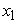through. As an example, to find the first row of A2, we add the 273 at position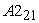and the 273 at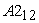from the larger matrix A. Those values are stored as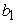. A21,1 is treated as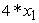based on our choices in delta x and delta y, A22,3 isand A22,1 isin the row below. Our full system looks like this.Before we move on to the coding of the problem into MATLAB, let’s first discuss how the relaxation factor was chosen. If A is a positive definite matrix and, then the SOR is guaranteed to converge for any initial choice of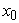. If in addition A is tridiagonal and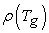where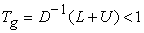then the optimal choice of the relaxation factor is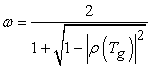which is how we obtained our optimum relaxation factor, 1.1805. All of our pieces are now set, let’s put it all together in MATLAB. Running the code through, the x vector’s last iteration is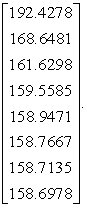If we compare that with the exact results ofwe getand if we compare the errors as decimals, we get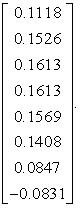Compare the times. Running just the SOR through MATLAB, it takes 0.084 seconds from the setup of the matrix to displaying the results at the very end of it while MATLAB’s built in functions can solve the exact answer in 0.020 seconds. While that is much faster, bear in mind SOR does not need to have the inverse of matrix A, which that alone can make a world of difference in deciding on which method to solve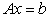. SOR can also be a very nice system to use if matrix A is very large and sparse. Storing all of those zeros can be a waste of space in the computer as it slows down computation times.

### 4. Conclusion and Future Work

In this paper, we have highlighted the importance of using SOR interactive solve method for accelerating solution of real word problems.It should come as no surprise the SOR method is an industry standard for solving matrix systems. Further research will be needed to find an SOR algorithm that would produce better, closer results to the exact values. That being said, where the SOR method shines through lays in its speed and its ability to solve any n x n system without the need of the coefficient matrix A having an inverse and without the need to store the matrix entirely, saving space and time.

### Acknowledgement

It is with great pleasure that we publish this paper first and foremost and our sincere thank and appreciation to MATH674 course (Spring 2016) instructor Dr. Ruijun Zhao, Associate Professor Mathematics, Department of Mathematics and Statistics, Minnesota state university, Mankato, USA for his tremendous assistance and valuable guidance.

### References

  A. Hadjidimos, “Successive Overrelaxation (SOR) andrelated methods,” Journal of Computational and Applied Mathematics, vol. 123, no. 1, pp. 177-199, 2000.In article View Article  D. Xie, “A new block parallel sor method and its analysis,” SIAM Journal on Scientific Computing, vol. 27, no. 5, pp. 1513-1533, 2006.In article View Article  Ioannis K Argyros (2000) BACK MATTER. Advances in the Efficiency of Computational Methods and Applications: pp. 503-546.In article View Article  O. Mangasarian and D. Musicant, “Successive Overrelaxation for support vector machines,” Neural Networks, IEEE Transactions on, vol. 10, no. 5, pp. 1032-1037, 1999.In article View Article  PubMed  Ortega, J. M., NumericalAnalysis; A Second Course, Academic Press, New York, 1972, 201 pp.In article  Richard L. Burden and J. Douglas Faires, 2010: Numerical Analysis 9th edition, Brooks-Cole CENGAGE Learning, 895 pgs.In article  Ronald S. Besser, 2002, Spreadsheet Solutions to Two- Dimensional Heat Transfer Problems, 6 pp.In article  Shi-Liang Wu and Yu-Jun Liu, A New Version of the Accelerated Overrelaxation Iterative Method, Hindawi Publishing Corporation Journal of Applied Mathematics, Volume 2014, Article ID 725360, 6 pagesIn article  Sparsh Mittal, “A Study of Successive Overrelaxation Method Parallelization Over Modern HPC Languages”, International Journal of High Performance Computing and Networking archive Volume 7 Issue 4, June 2014 Pages 292-298In article  Wikipedia, 2016: Successive Over-relaxation [https://en.wikipedia.org/wiki/Successive_over-relaxation].In article

### Appendix

The code itself

clear all;

clc;

format short;

A = [273 273 273 273 273 273 273 273 273 273; 273 0 0 0 0 0 0 0 0 273; 273 0 0 0 0 0 0 0 0 273;

273 0 0 0 0 0 0 0 0 273 ; 273 0 0 0 0 0 0 0 0 273; 273 0 0 0 0 0 0 0 0 273 ; 273 0 0 0 0 0 0 0 0 273;

273 0 0 0 0 0 0 0 0 273; 273 0 0 0 0 0 0 0 0 273; 273 273 273 273 273 273 273 273 273 273]

k = length(A);

A2 = zeros(k-2);% Inner most set of zeros, in this case, 8 x 8.

m = length(A2);

b = zeros(8,1); % Seed for the b vector.

dx = 1;

dy = 1;

for a = 1:m;

A2(a,a) = (2/(dx^2)) + (2/(dy^2)); % Sets the main diagonal of A2.

end

for c = 1:(m-1)

A2(c,c+1) = (-1)/(dx^2); % Sets the off diagonals of A2 = -1.

A2(c+1, c) = (-1)/(dy^2); % Had to do it this way because it added rows and collums, giving us a 9x9.

end

b = [546 273 273 273 273 273 273 546]';

condnum = norm(A2) * norm(inv(A2))

D = diag(diag(A2)); % Matrix with only the values of the diagonal of A2.

F = tril(-A2,-1); % " " values of the lower triangular matrix of A2.

E = triu(-A2,1); % Upper triangular part.

Tj = inv(D * (F+E));

rho_Tj = max(abs(eig(Tj)));

% omega = 1.1; % The relaxation factor, must be > 1. Use 1.1

omega = 2/(1+sqrt(1-rho_Tj^2));

fi = [273; 273; 273; 273 ;273; 273 ;273 ;273]; % Initial guess.

fori = 1:m

sigma = zeros(m,1);

for j = 1:m

ifi ~= j % ~= is "not equal to".

sigma = sigma + (A2(i,j)*fi(j));

end

end

fi(i) = (1-omega)*fi(i) + (omega/A2(i,i)*(b(i)-sigma(i)));

end

fi

exac = inv(A2) * b;